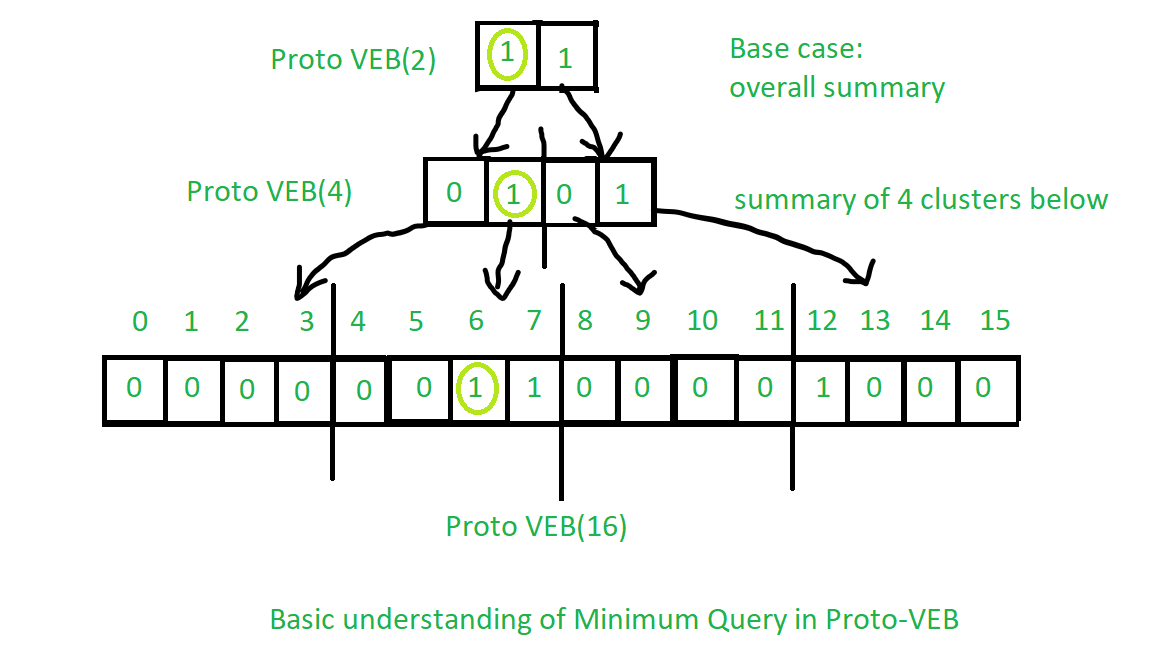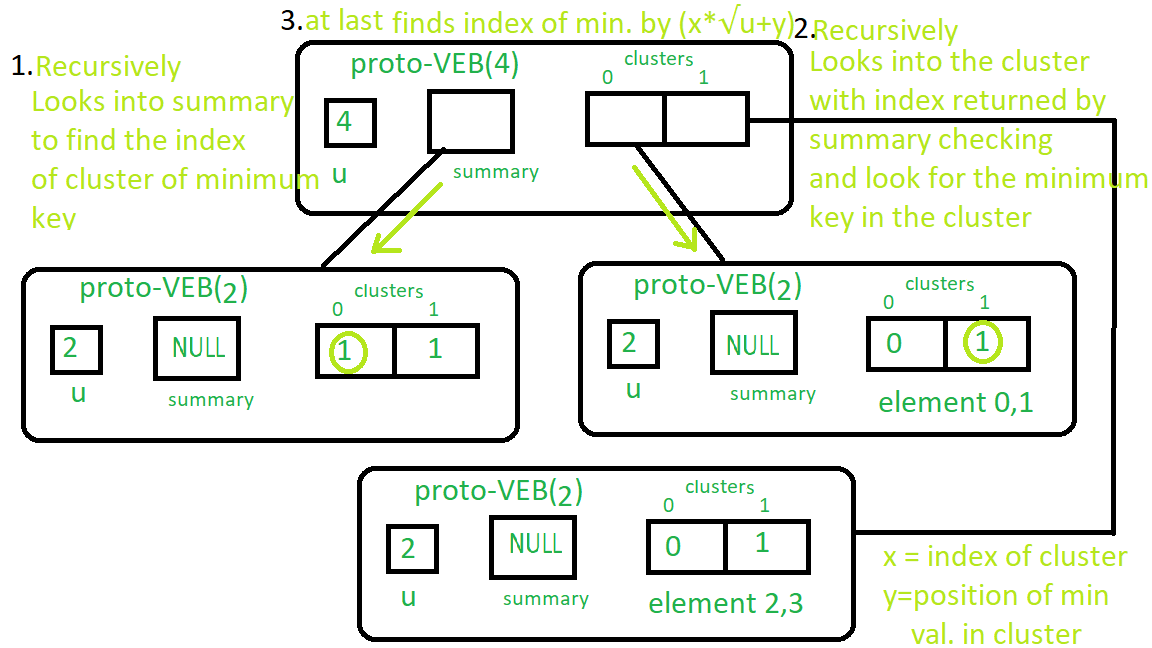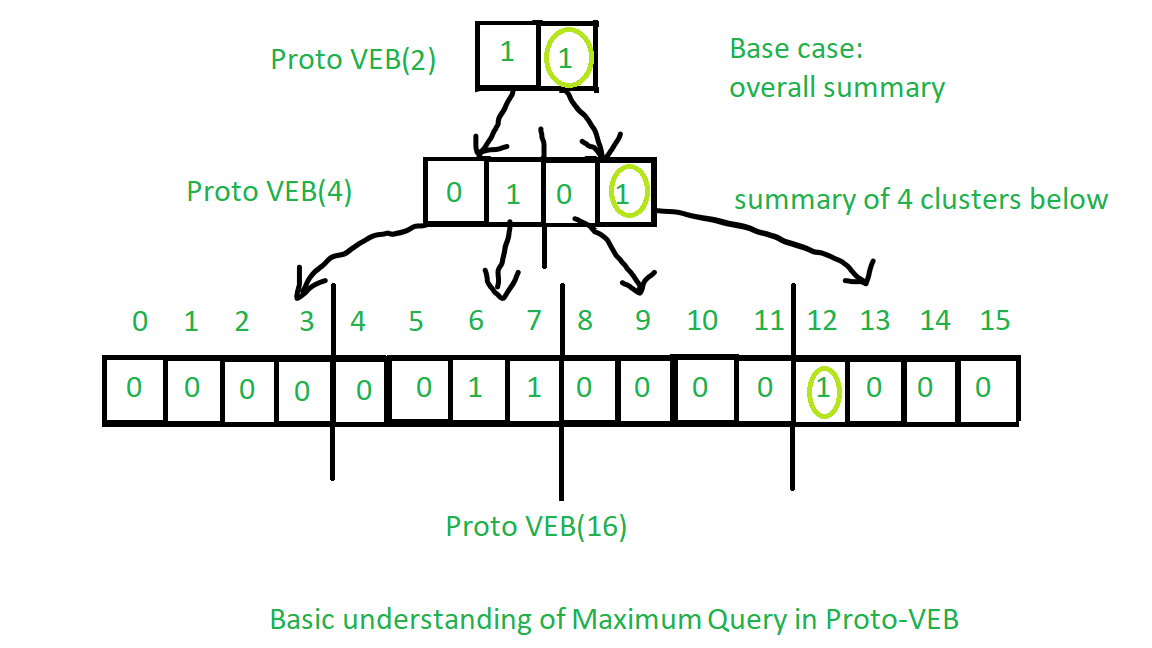# Proto Van Emde Boas Tree | Set 5 | Queries: Minimum, Maximum

Please check previous sets of Proto Van Emde Boas Tree article first. It is highly recommended.

Procedure for finding minimum:

1. Base Case: If the size of Proto-VEB is 2 then we will return smallest key present in the cluster if no keys present then we will return -1 as the symbol that no keys are present.
2. Recursion:
• We will start recursion over summary until we reach the first true value(In the code, first not nullptr in the summary cluster) which shows that there is a key present in that cluster.
• Now We will find the position of the key in that cluster using again recursively call over a cluster to find the first true value (In the code, first not nullptr in the cluster) in a cluster as we have done above.
• Finally, we will return the index of that key according to cluster number we get from procedure over summary and position we get from the procedure over the cluster in the last step.

See the image below for the basic understanding of the operation:

Observe the light green circles from top to bottom:See the image below for real Proto – VEB minimum operation:

Follow the instructions in order of numberings.You can easily get the idea of Maximum from the minimum procedure. See the image below:

Observe the light green circles from top to bottom:Implementation of the above algorithm:

 // C++ implementation of the approach  #include  using namespace std;     class Proto_Van_Emde_Boas {  public:      // Total number of keys      int universe_size;         // Summary      Proto_Van_Emde_Boas* summary;         // Clusters array of Proto-VEB pointers      vector clusters;         int root(int u)      {          return int(sqrt(u));      }         // Function to return cluster numbers      // in which key is present      int high(int x)      {          return x / root(universe_size);      }         // Function to return position of x in cluster      int low(int x)      {          return x % root(universe_size);      }         // Function to return the index from      // cluster number and position      int generate_index(int cluster, int position)      {          return cluster * root(universe_size) + position;      }         // Constructor      Proto_Van_Emde_Boas(int size)      {          universe_size = size;             // Base case          if (size <= 2) {                 // Set summary to nullptr as there is no              // more summary for size 2              summary = nullptr;                 // Vector of two pointers              // nullptr in starting              clusters = vector(size, nullptr);          }          else {                 // Assiging Proto-VEB(sqrt(u)) to summary              summary = new Proto_Van_Emde_Boas(root(size));                 // Creating array of Proto-VEB Tree pointers of size sqrt(u)              // first all nullptrs are going to assign              clusters = vector(root(size), nullptr);                 // Assigning Proto-VEB(sqrt(u)) to all its clusters              for (int i = 0; i < root(size); i++) {                  clusters[i] = new Proto_Van_Emde_Boas(root(size));              }          }      }  };     // Function that returns true if the  // key is present in the tree  bool isMember(Proto_Van_Emde_Boas* helper, int key)  {         // If key is greater then universe_size then      // returns false      if (key >= helper->universe_size)          return false;         // If we reach at base case      // the just return whether      // pointer is nullptr then false      // else return true      if (helper->universe_size == 2) {          return helper->clusters[key];      }      else {             // Recursively go deep into the          // level of Proto-VEB tree using its          // cluster index and its position          return isMember(helper->clusters[helper->high(key)],                          helper->low(key));      }  }     // Function to insert a key in the tree  void insert(Proto_Van_Emde_Boas*& helper, int key)  {      // If we reach at base case      // then assign Proto-VEB(1) in place      // of nullptr      if (helper->universe_size == 2) {          helper->clusters[key] = new Proto_Van_Emde_Boas(1);      }      else {             // Recursively using index of cluster and its          // position in cluster          insert(helper->clusters[helper->high(key)],                 helper->low(key));             // Also do the same recusion in summary VEB          insert(helper->summary, helper->high(key));      }  }     // Function to return the minimum key from the tree  int minimum(Proto_Van_Emde_Boas* helper)  {      // Base case choses the least key      // present in the cluster      if (helper->universe_size == 2) {          if (helper->clusters) {              return 0;          }          else if (helper->clusters) {              return 1;          }             // No keys present then return -1          return -1;      }      else {             // Recursively find in summary for          // first 1 present in Proto-VEB          int minimum_cluster = minimum(helper->summary);          int offset;             // If no key is present in          // the cluster then return -1          if (minimum_cluster == -1) {              return -1;          }          else {                 // Recursively find the position of the key              // in the minimum_cluster              offset = minimum(helper->clusters[minimum_cluster]);                 // Returns overall index of minimum key              return helper->generate_index(minimum_cluster, offset);          }      }  }     // Function to return the maximum key from the tree  int maximum(Proto_Van_Emde_Boas* helper)  {         // Return the maximum key present in      // the cluster      if (helper->universe_size == 2) {          if (helper->clusters) {              return 1;          }          else if (helper->clusters) {              return 0;          }             // Return -1 if no keys present in the          // cluster          return -1;      }      else {             // Recursively find the last 1 present          // in the summary          int maximum_cluster = maximum(helper->summary);          int offset;             // If no key is present in          // the cluster then return -1          if (maximum_cluster == -1) {              return -1;          }          else {                 // Recursively find the position of the key              // in the maximum_cluster              offset = maximum(helper->clusters[maximum_cluster]);              return helper->generate_index(maximum_cluster, offset);          }      }  }     // Function to delete a key from the tree  void pveb_delete(Proto_Van_Emde_Boas*& helper, int key)  {         // Base case: If the key is present      // then make it nullptr      if (helper->universe_size == 2) {          if (helper->clusters[key]) {              delete helper->clusters[key];              helper->clusters[key] = nullptr;          }      }      else {             // Recursive delete to reach at the base case          pveb_delete(helper->clusters[helper->high(key)], helper->low(key));             bool isanyinCluster = false;             // Iterate over the cluster of keys to check whether          // any other key is present within that cluster          // If yes then we should not update summary to 0          // else update summary to 0          for (int i = helper->high(key) * helper->root(helper->universe_size);               i < (helper->high(key) + 1) * helper->root(helper->universe_size);               i++) {                 // If member is present then break the loop              if (isMember(helper->clusters[helper->high(key)], i)) {                  isanyinCluster = true;                  break;              }          }             // If no member is present then          // update summary to zero          if (isanyinCluster == false) {              pveb_delete(helper->summary, helper->high(key));          }      }  }     // Driver code  int main()  {      Proto_Van_Emde_Boas* hello = new Proto_Van_Emde_Boas(4);         cout << boolalpha;         insert(hello, 2);         insert(hello, 3);         cout << minimum(hello) << endl;         cout << maximum(hello) << endl;  }

Both Minimum and Maximum query runs in O(log2(u)) time complexity.

Recurrence Relation:

T(u) = 2T() + O(1)

My Personal Notes arrow_drop_upI am doing BTech at Dhirubhai Ambani Institute of Information and Communication Technology

If you like GeeksforGeeks and would like to contribute, you can also write an article using contribute.geeksforgeeks.org or mail your article to contribute@geeksforgeeks.org. See your article appearing on the GeeksforGeeks main page and help other Geeks.

Please Improve this article if you find anything incorrect by clicking on the "Improve Article" button below.